# Insertion In Red-Black Trees

## Introduction

A red-black tree is one type of binary search tree that satisfies the following properties:

•  Every node is either red or black.
• The root is black.
• Every leaf (nil) is black.
• If a parent node is red, then both of its children are black.
•  All simple paths from the node to descendant leaves contain the same number of black nodes for each node.

In a red-black tree, every new node is inserted with the color red. The insertion in the red-black tree is similar to the insertion operation in a binary search tree. But nodes are inserted with a color property. After insertion operation, we need to check all the properties of the red-black tree. If all the properties are not satisfied, we perform the following operation to make it a red-black tree.

• Recolor
• Rotation followed by recolor.

## Algorithm

Step 1: Check if the tree is empty.

Step2: If the tree is empty when inserting the new node as root node with color black an exit from the operation.

Step3: If the tree is not empty, insert the new node as a leaf node with the red color.

Step4: If the parent of the new node is black, then exit from the operation.

Step5: If the new node's parent is red, change the color of the parent node's sibling to the new node.

Step6: If it is colored black or null, then make a suitable rotation and recolor it.

Step7: If it is colored red, then perform a recolor. Repeat the same until the tree becomes a red-black tree.

Let’s visualize this through an example.

• Let the new node be :

• Let y be the leaf (i.e., nil) and x be the root of the tree. The new node will be inserted in the following tree.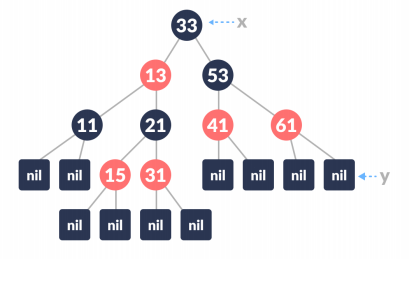source

• Check if the tree is empty (i.e., whether x is nil). If yes, insert the new node as a root node and color it black.

• Else, repeat steps following steps until a leaf (nil) is reached.

1. Compare the key of the new node with the root's key.

2. If the new key is greater than the root's key, traverse through the right subtree.

3. Else traverse through the left subtree.

So, the new node can be inserted as the right child of the node with key=15.

• If the leaf node Key is greater than the key of the new node, make the new node as the right child.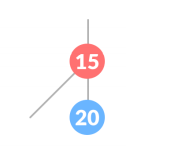source

• Assign NULL to the left and right child of the new node and make it red-colored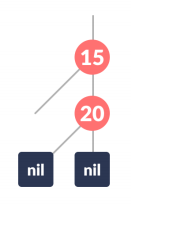source

• Call Insert Fix-algorithm to maintain the property of the red-black tree if violated after insertion.

Insert Fix():

Algorithm to Maintain Red-Black Property After Insertion

• While the parent p of the new node is RED, do the following.

• do the following if p is the left child of grandParent gP of the new node

Case-I

1. If the color of the right child of gP(grandparent of the new node) is RED, then make the color of both the children of gP as BLACK and the color of gP as RED.
2. Assign gP as newNode.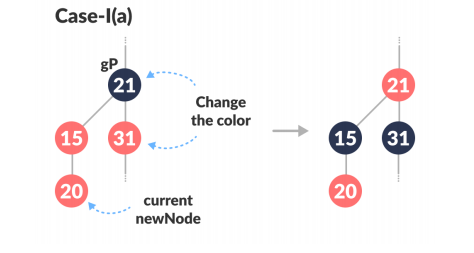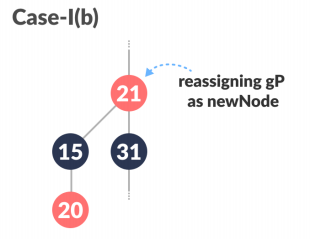source

Case-II

1. Else if the new node is the right child of p then, assign it as the new node.
2. Left-Rotate the new node.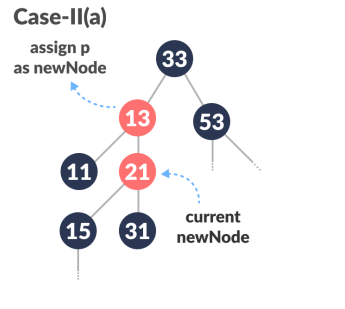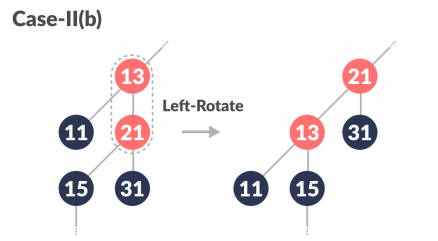source

Case-III:

1. Set the color of p as BLACK and the color of gP as RED.
2. Right-Rotate the gP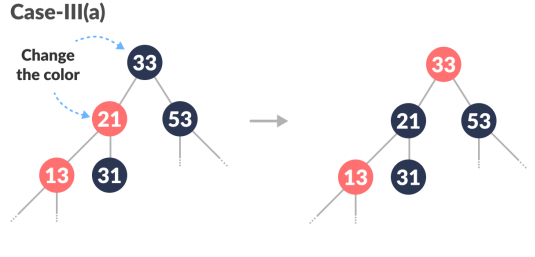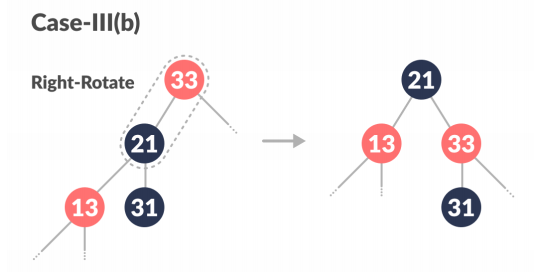source

• Else, do the following :
1. If the color of the left child of gP of the new node is RED, recolor the color of gP as RED and the color of both the children of gP as BLACK.
2. Assign gP as the newNode.
3. Else if the new node is the left child of p then, assign p to newNode and Right-Rotate the newNode.
4. Recoloring, i.e., the color of p as BLACK and the color of gP as RED.
5. Left-Rotate the gP.

• Make the root of the tree as BLACK.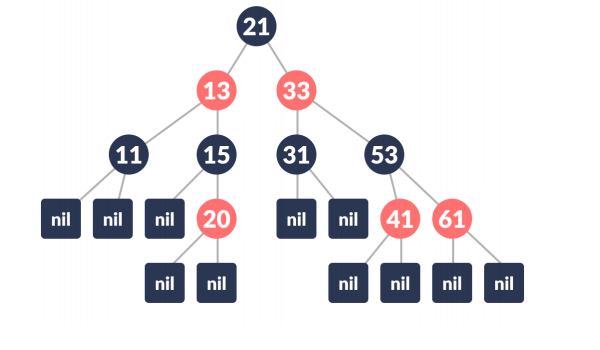source

It is the final red-black tree after insertion.

Structure of the red-black tree node

## Complexity analysis

There is only a single while loop in the insertion algorithm. In that loop, the node at the root of the sub-tree whose red-black property we are trying to restore, x, may be moved up the tree at least one level in each iteration of the loop. Since the balanced red-black tree has O(log n) height, there are O(log n) iterations. The tree_insert routine also has O(log n) complexity, so overall, the rb_insert() function also has O(log n) complexity.

## FAQs

### 1. What is the red-black tree?

A red-black tree is one type of binary search tree that satisfies the following properties:

•  Every node is either red or black.
• The root is black.
• Every leaf (nil) is black.
• If a parent node is red, then both of its children are black.
•  All simple paths from the node to descendant leaves contain the same number of black nodes for each node.

### 2. What is the color of the root of a red-black tree?

The root node of a red-black tree is always black.

### 3. What is the height of a red-black tree?

As the red-black tree is one type of balanced tree, the height of the N node red-black tree is logN.

### 4. What is the application of a red-black tree?

It is used for implementing TreeSet, TreeMap in java and set, map in C++ STL library.

### 5. What color do we assume while inserting a new node?

We insert a new node as a red node, then recolor it if needed to satisfy the red-black tree properties.

## Key Takeaways

This article covered how to make insertion in the Red-Black Trees. We have learned about the algorithm to insert a node in the Red-Black Tree and restore its property after insertion.

Side by side, we should also learn about searching and deletion operations in the Red-Black Tree.

Apart from this, you can practice a wide range of coding questions commonly asked in interviews in CodeStudio. Along with coding questions, we can also find the interview experience of scholars working in renowned product-based companies here.

Happy learning!